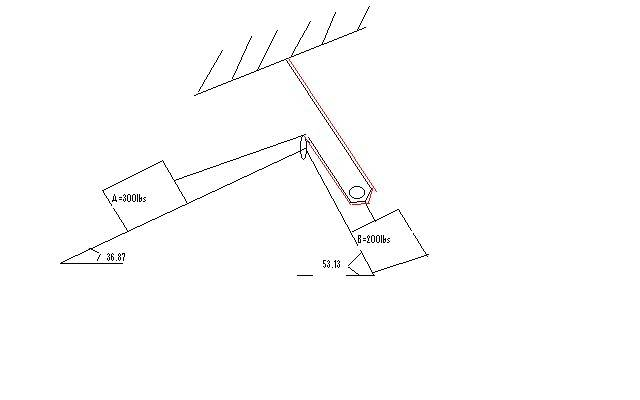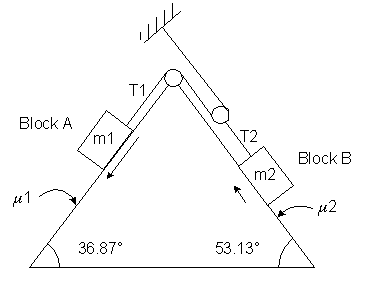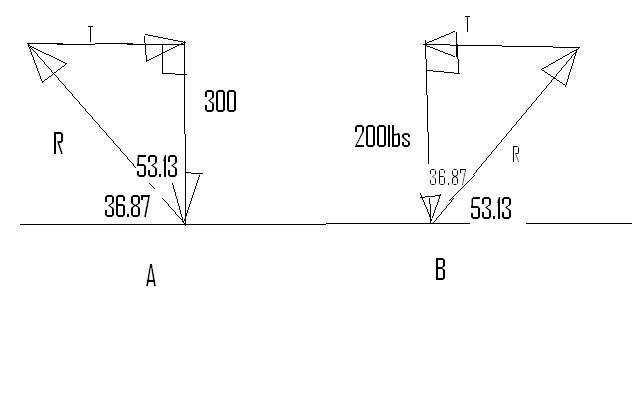# Newtons law

## Homework Statement

Homework Statement [/b]

am i right? please help me .. thnx

Homework Statement
the blocks shown in the figure are connected by flexible, inextensible cords passing over frictionless pulleys. at A, the coefficient fiction is 0.30 while at B, it is 0.40. compute the magnitude and direction of the friction forces and each block.## Homework Equations

for block A=
T-mgsin53.13-Fmax=0
Fmax=0.3(300cos53.13)

for block B=
T-mgsin36.87-Fmax=0
Fmax=0.40(200cos36.87)

## The Attempt at a Solution

for A:

Fmax=μk(m1)gcos53.13
54=μk(m1)gcos53.13
μk=0.03

T-300(9.81)sin53.13-μk(300)9.81cos53.13=(300)a eq(1)
200g-T=200a eq(2)

subtitute (1) and (2) to find a

a=-3.79

for block B:

Fmax=μk(m2)gcos36.87
64=μk(m2)gcos36.87
μk=0.04

T-200(9.81)sin36.87-μk(200)9.81cos36.87=(200)a eq(1)
300g-T=300a eq(2)

subtitute (1) and (2)

to find a

a=0.2

AM i DOiNG RiGHT ?? please comment .. im lack of knowledge ..

## Answers and Replies

help me please ! im confuse what would be the fomula il use

gneill
Mentor
A couple of things. First, the weights of the blocks are specified in pounds (lbs), so unless you convert to Newtons (and kilograms for mass) you can't use g = 9.81 m/s2 because the units won't match. Perhaps you might consider switching the units to kg and N before proceeding, or use g = 32.2 ft/s2 and associated other units of the English system in your calculations.

Second, before writing the formulas that will describe the Free Body Diagrams for each block you should determine which (if any) block will rise and which will fall. This will let you ascertain the directions to assign to the frictional forces. To do this, imagine that you hold the 300lb block in place and banish all friction. The system will be held static by your holding that block in place. What is the tension in the rope attached to the 300lb block? Is it sufficient to move the block upward on its slope when you release it, or will it want to fall?

thnx .. block A = 136.08 kg
B=90.72 kg

i already draw the free body block A will fall and block be will go upward..

the motion going to left.
what would be posible formula to use in this problem? are my solution right?

Last edited:
i need to know if my formula is right or wrong? f wrong what part? or at begining im wrong ? what wod be the possible formula i used,,, thnx alot

gneill
Mentor
I don't see where you've accounted for the fact that the accelerations of the two blocks will be different (thanks to the pulley geometry) and how the tensions in the two ropes will be different (again thanks to the pulley geometry). How are they related?

With these relationships in hand, if you then write equations for the two accelerations you'll have sufficient information to solve the simultaneous equations.

i solve it again through dis solution..

so :

T-m1gsin53.13-μkm1gcos53.13=m1a
eq 1

T-505.44=90.72a

eq2

T-m2gsin36.87-μkm2gcos36.87=m2a

so T=577.27
a=-3.42

for 2nd block:

T-m2gsin36.87-μkm2gcos36.87=m2a

eq 1 : T-505.50=90.72a

T-m1gsin53.13=μkm1gcos53.13=m1a

eq2 : T-1043.93=136.08a

so T=571.36

a=0.73

am i doing right?? please help

gneill
Mentor
No, it doesn't look right to me. You're still not relating the separate accelerations and tensions via the pulley constraints. Also, I can't understand why you'd be writing two FBD equations for each block and using the properties of the other block's situation to do so (its slope angle and frictional coefficient).

Write one FBD equation for each block. It will encompass just the forces acting on that block. For block 1 leave the tension as T1 and the acceleration as a1. Do the same for block 2, but here the tension is T2 and the acceleration a2.

Then write down the relationships that pulley enforces on a1 and a2, and on T1 and T2. Use these relationships as two more equations. Altogether you'll have four equations: two from the FBD's for the blocks and the two 'pulley relationship' equations. Simple substitution should allow you to solve for the accelerations and tensions.

sory im weak here in physics ..

hihi! this?
μm1g-T1=m1a1
m2gsin(teta)-T2=m2a2

so

a=(g(μm1-m2))/m1+m2

last try .. hihihi sorry ..

gneill
Mentor
sory im weak here in physics ..

hihi! this?
μm1g-T1=m1a1
m2gsin(teta)-T2=m2a2

so

a=(g(μm1-m2))/m1+m2

last try .. hihihi sorry ..

Nope.

Why don't you focus on just one block for now. Take the left hand one, with mass m1 sitting on a slope of $\theta 1 = 36.87°$, friction coefficient $\mu1$, and with rope tension T1. That should be sufficient information to write the one FBD equation.

Last edited:
for A:

T1=136.08sin53.13/sin36.87

B:

T2=90.72sin36.87/sin53.13

maybe il copy later im weak .. grr!!! sorry sir thnx for intertaining

for block A

Fmax=0.30(136.08)cos53.13
Fmax=24.5
T=300(9,81)sin53.13
T=2354.4

For B
Fmax=0.4(90.72)cos36.78
Fmax=21.77
T=90.72sin36.87
T=1177.20

is this right?

gneill
Mentor
No. I don't understand why you're mixing angles from the opposite blocks in the equations for a given block. For example, for block A, the 136kg block sitting on the 36.87° slope, why do you have cos(53.13) and sin(53.13) in its FBD equation? When you write the equation for one block, consider only the forces and conditions that apply to that one block. For block A leave the acceleration as a1 and the tension as T1. DO NOT TRY TO FIND NUMERICAL RESULTS FOR THEM AT THIS STAGE! You don't have enough information yet. Note that a1 DOES NOT equal a2, and T1 DOES NOT equal T2.

You cannot solve for the accelerations and tensions until you have resolved the pulley's influence: You must state the relationships between the accelerations and tensions that the pulley creates.

for A:
T-m1gsin53.13-μkm1gcos53.13=m1a
m1g-T=m2a

(T-1065.5)/136.08=a
(1334.94-T)=90.72a

T=1227.4
a=1.19

hehe! im trying .. my memory is low :)

last attempt to try .. hihi

Last edited:
gneill
Mentor
The accelerations of the blocks are not the same. You must use separate variables for each. The tensions in the ropes attached to each block are not the same. You must use separate variables for each.

Use a1, T1, a2, T2 for these variables. Write the FBD equations for each block (separately) using these variables for the unknowns. Do not try to combine the equations yet. You might want to use symbols only for now (no numerical values). In which case, use u1 and u2 for the friction coefficients and q1 and q1 for the angles.

Here's a new diagram that may help:#### Attachmentsteta=tan^-1(.3)
teta=16.70

sine law:
T/sin53=136.08/sin36.87
T=181

B::
teta=tan^-1(.4)
teta=21.80

is this right?

T/sine36.87=90.72/sine53.13
T=68.04

Last edited:
PeterO
Homework Helperteta=tan^-1(.3)
teta=16.70

sine law:
T/sin53=136.08/sin36.87
T=181

B::
teta=tan^-1(.4)
teta=21.80

is this right?

T/sine36.87=90.72/sine53.13
T=68.04

Diagrams in last two posts represent the two angles like 45 degrees, so no hints as to which functions and angles you should be using.

Did you recognise that the original situation is based on a 3-4-5 triangle, so the sine and cosine values are easy to compute.

Yes, the frictional force in the given problem, as presented, is independent of acceleration or velocity of either block, but only dependent upon the direction of the normal force for each.

However, *as presented*, the velocity of each block is unknown, and therefore the direction of the direction of the frictional force is ambiguous.

gneill
Mentor
Yes, the frictional force in the given problem, as presented, is independent of acceleration or velocity of either block, but only dependent upon the direction of the normal force for each.

However, *as presented*, the velocity of each block is unknown, and therefore the direction of the direction of the frictional force is ambiguous.

This issue was (apparently) dealt with during the first few posts of the thread. The OP decided that block A is moving downwards on its slope, thus block B is moving upwards.

PeterO
Homework Helper
help me please ! im confuse what would be the fomula il use

The problem as you stated was:

compute the magnitude and direction of the friction forces and each block.

The maximum size of the friction forces on the two blocks are 72lb [on the 300lb block] and 48lb [on the 200lb block].

Since, as you correctly stated the blocks will be moving to the left, the maximum friction force should apply - and in a direction opposed to the motion.

so 72lb up the left hand slope, and 48lb down the right hand slope.

It would probably be nice from a physics point of view to work the answer in SI units, but given that information was given in the American version of British Units, why not answer in the American form of British Units.
[Note: in British/Imperial units lb is a unit of mass. In the American form lb is a unit of Force apparently]

gneill
Mentor
compute the magnitude and direction of the friction forces and each block.

I suspect that there are some missing words in that statement. Possibly it should read:

"Compute the magnitude and direction of the friction forces and acceleration of each block."

The OP should clarify this.

PeterO
Homework Helper
I suspect that there are some missing words in that statement. Possibly it should read:

"Compute the magnitude and direction of the friction forces and acceleration of each block."

The OP should clarify this.

The acceleration will be exceptionally small, as I suspect the situation is within 4 lb of balance.